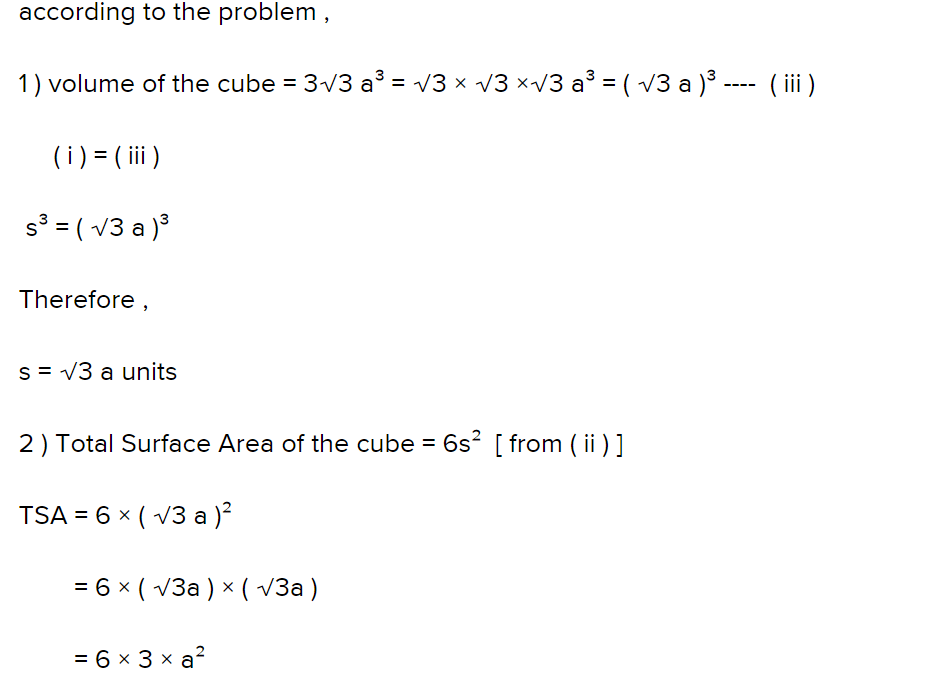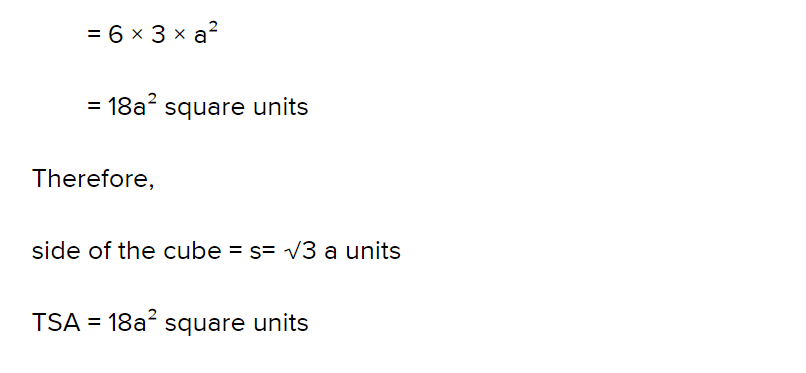# Test: Volume of a Cuboid

## 20 Questions MCQ Test NCERT Mathematics for CAT Preparation | Test: Volume of a Cuboid

Description
Attempt Test: Volume of a Cuboid | 20 questions in 20 minutes | Mock test for Class 9 preparation | Free important questions MCQ to study NCERT Mathematics for CAT Preparation for Class 9 Exam | Download free PDF with solutions
QUESTION: 1

Solution:
QUESTION: 2

Solution:
QUESTION: 3

### Four cubes each of side 5 cm are joined end to end. The volume of the resulting cuboid is​

Solution:
QUESTION: 4

A cubical tank has an edge equal to 6 m. The amount of water it will contain when it is one-third filled is

Solution:
QUESTION: 5

The lateral surface area of a cube is 100 m2. The volume of the cube is:​

Solution:
QUESTION: 6

A rectangular tank has dimensions 4 m × 3 m × 3 m. The amount of water it will contain when it is two-third filled is

Solution:
QUESTION: 7

Three cubes of side a are joined end to end. What is the surface area of the new solid thus formed?​

Solution:
QUESTION: 8

The capacity of the tank of dimensions 7 m × 7 m × 5 m is​

Solution:
QUESTION: 9

Find the volume of a cubical box whose surface area is 13.50 cm2.​

Solution:
QUESTION: 10

A cubical tank has base area of 49 m2 and is half filled. The amount of water it can hold is

Solution:

A cube has all three sides equal.

let the side be a

so base area = a2 = 49

a = 7 m

and it is half filled

so volume of water in it= volume it can hold more

= base area x height

= 49 x 7/2

=171.5 m3

1m= 1000L

so amount of water it can hold = 171.5 x 1000 = 171500 L

QUESTION: 11

The volume of a cube is 343 cm3. Its surface area would be​

Solution:
QUESTION: 12

The volume of a cylinder of altitude 15 cm is 1200 cm3. The area of its base is​

Solution:
QUESTION: 13

The volume of water in a cuboidal container of dimensions 50 cm, 20 cm and 30 cm will be

Solution:
QUESTION: 14

If the volume of a cube is 3√3a3, then total surface area of the cube is

Solution:QUESTION: 15

Seven cubes each of sides 4 cm are joined end to end. The volume of the resulting cuboid would be​

Solution:
QUESTION: 16

A measurement box measures 18 cm × 6 cm × 2 cm. The volume of the packet containing 12 such boxes is​

Solution:
QUESTION: 17

Find the edge of a cube of volume equal to the volume of a cuboid of dimensions 24 cm × 12 cm × 6 cm

Solution:
QUESTION: 18

The areas of three adjacent faces of a cuboid are a, b and c. If its volume is V, then V =

Solution:
QUESTION: 19

Find the number of bricks, each measuring 25 cm long, 12.5 cm high and 7.5 cm thick required to construct a wall 6 m long, 5 m high and 0.5 m thick, while the cement and sand mixture occupies ignored.​

Solution:
QUESTION: 20

A cube of edge 4 cm is converted to a cuboid of height 4 cm, so the area of the base of the cuboid must be​

Solution:Use Code STAYHOME200 and get INR 200 additional OFF Use Coupon Code